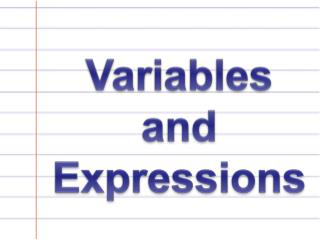Download PresentationVariables and Expressions

# Variables and Expressions - PowerPoint PPT PresentationDownload Presentation## Variables and Expressions

- - - - - - - - - - - - - - - - - - - - - - - - - - - E N D - - - - - - - - - - - - - - - - - - - - - - - - - - -
##### Presentation Transcript

1. Variables and Expressions

2. Standards and Objectives GLE 0506.3.2 – Use variables appropriately to represent numbers whose values are not yet known. Objectives: The students will translate words into algebraic expressions and algebraic expressions into words.

3. Variable: A letter or symbol that represents an unknown amount that can vary or change. Example: 5+n In 5+ n, “n” is the variable.

4. Algebraic Expression: A mathematical phrase involving variables, numbers, and operations. 3+n=7 is an Algebraic Expression 3+4=7 is NOT an Algebraic Expression

5. Math Words to Review… • Difference: The number that results from subtracting one number from another. • Product: The number that is the result of multiplying two or more factors. • Quotient: The number other than the remainder that is the result of dividing. • Sum: The number that is the result of adding two or more addends.

6. What are some ways we can say this numerical expression? 3 + 4 Three plus Four Four more than Three The sum of Three and Four

7. What are some ways we can say this numerical expression? 7 - 2 The difference of Seven and Two Two less than Seven Seven minus Two

8. But how can we say this...

9. 5 + 5 +

10. How do you read this expression? 5 + • This ALGEBRAIC EXPRESSIONcan be read as: • The sum of five and a number • Five more than a number

11. What about this one? How do we read this ALGEBRAIC EXPRESSION? 4 X • The product of four and a number • Four times a number

12. In order to write a mathematical expression for the word phrase “5 plus a number” you must use a VARIABLE. • A variable is a letter or symbol that you use to represent unknown values that can vary or change. • When an expression includes a variable, it is called and ALGEBRAIC EXPRESSION. LET’S PRACTICE…

13. Translate these Algebraic Expressions into Words… n + 9 A NUMBER plus nine.

14. Translate these Algebraic Expressions into Words… n ÷ 12 A NUMBER divided by twelve.

15. Translate these Algebraic Expressions into Words… 4 - n Four minus a NUMBER. Or A NUMBER less than 4.

16. Translate these Algebraic Expressions into Words… 8 X n Eight multiplied by a NUMBER. Or The product of eight and a NUMBER.

17. Now, let’s try writing each word phrase as an ALGEBRAIC EXPRESSION. Add six to a number. n + 6 Subtract a number from ten. 10 – n

18. Write each word phrase as an ALGEBRAIC EXPRESSION. The product of nine and a number. 9 X n OR n X 9 Fifteen divided by a number. 15 ÷ n

19. Write each word phrase as an ALGEBRAIC EXPRESSION. Twelve less than a number. n - 12 A number added to nine. 9 + n

20. Time for some TEAM WORK!

21. NOW IT’S YOUR TURN! Page 146 of your Math text book. Problems 1-20.

22. Standards and Objectives GLE 0506.3.2 – Use variables appropriately to represent numbers whose values are not yet known. Objectives: The students will translate words into algebraic expressions and algebraic expressions into words.

23. EXIT TICKET • Complete the following problems on your index card and turn in… • Translate this word phrase into an algebraic expression: • The product of a number and nine. • Translate this algebraic expression into a word phrase: • 10 - n